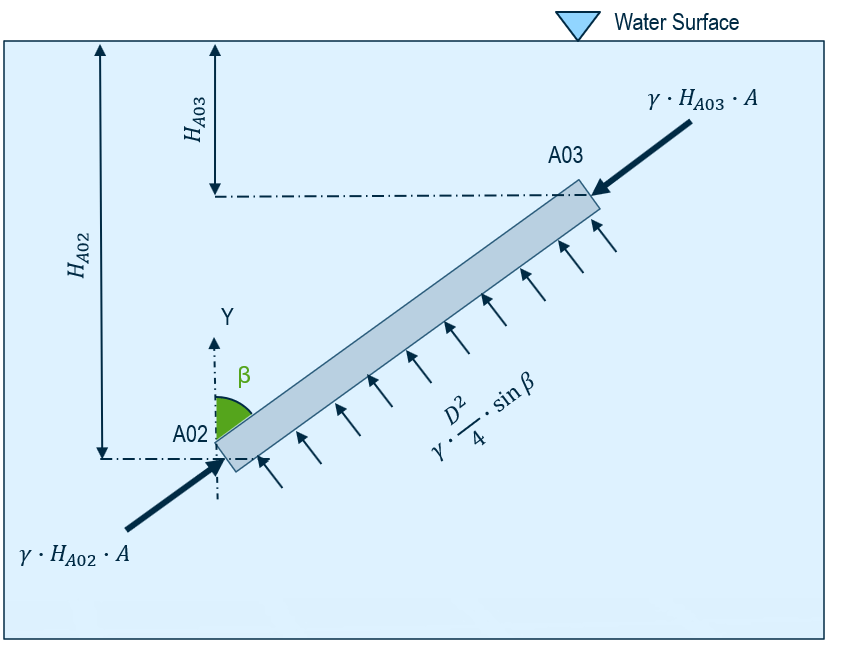# Buoyancy### Buoyancy

AutoPIPE calculate automatically the buoyancy force. Only the water level has to be set.

• AutoPIPE treats buoyant forces as a uniformly distributed load acting along the submerged length of pipe.
• End cap forces are considered by AutoPIPE for submerged pipes.
• Water surface elevation and other variables controlled through “Load > Buoyancy”.
• Suggested to have closely spaced nodes in the vicinity of water surface, preferably a node at water surface elevation.

### Modal Analysis

Natural frequencies of submerged or partially submerged structures would be lower than non-submerged structures.

This is due to mass of fluid required to be moved when structure moves.

Added mass coefficient (Ca) under Buoyancy dialog can be used to cater for the effects of added mass of fluid.

• For fully submerged pipes: $$M_{add} = C_a \times \rho \times A \times L$$
• For partially submerged pipes: $$M_{add} = C_a \times \rho \times A \times L_s$$